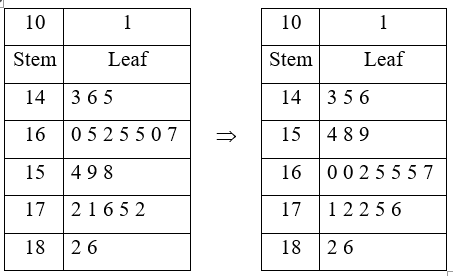Need Help?

Subscribe to Probability

###### \${selected_topic_name}
• Notes

Height of 20 students, in $\mathrm{cm}$ is given below
$\begin{array}{llll}{143} & {160} & {154} & {159} & {172} & {165} & {162} & {171} & {146} & {165} \\ {176} & {145} & {165} & {182} & {175} & {186} & {160} & {158} & {167} & {172}\end{array}$

a) Display the data in a stem-leaf plot.
b) Find the range of height.
c) What is the mean height?
d) What is the median height?
e) What percent of students having height greater than 175 $\mathrm{cm} ?$(b) range $=186-143=43 \mathrm{cm}$

(c) mean $=164.15 \ \mathrm{cm}$

$\frac{5 + 5}{2}=5$

(d) Median $=165 \ \mathrm{cm}$

(e) $\frac{3}{20}=15 \%$